# Civil Engineering - Applied Mechanics

### Exercise :: Applied Mechanics - Section 5

11.

A particle moving with a simple harmonic motion, attains its maximum velocity when it passes

 A. the extreme point of the oscillation B. through the mean position C. through a point at half amplitude D. none of these.

Explanation:

No answer description available for this question. Let us discuss.

12.

The moment of intertia of a circular lamina of diameter d, about an axis perpendicular to the plane of the lamina and passing through its centre, is

 A.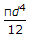B.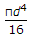C.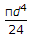D.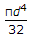E.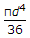Explanation:

No answer description available for this question. Let us discuss.

13.

The unit of impulse, is

 A. kg.m/sec B. kg.m/sec3 C. kg.m/sec2 D. kg.m2/sec.

Explanation:

No answer description available for this question. Let us discuss.

14.

If l is the span of a light suspension bridge whose each cable carries total weight (w) and the central dip is y, the horizontal pull at each support, is

 A.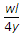B.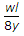C.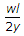D. wl.

Explanation:

No answer description available for this question. Let us discuss.

15.

The practical units of work, is

 A. erg B. joule C. Newton D. dyne.